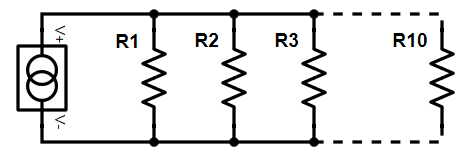# Current Divider CalculatorUse the calculator below to determine the current going through any branch in a parallel circuit.

Formula: Is = Itotal (Rtotal / Rn)

Enter a current source and resistance values to calculate the current through each resistor. You can calculate up to 10 branches.

Current Source:
A
R1:
Ω

A =
R2:
Ω

A =
R3:
Ω

A =
R4:
Ω

A =
R5:
Ω

A =
R6:
Ω

A =
R7:
Ω

A =
R8:
Ω

A =
R9:
Ω

A =
R10:
Ω

A =